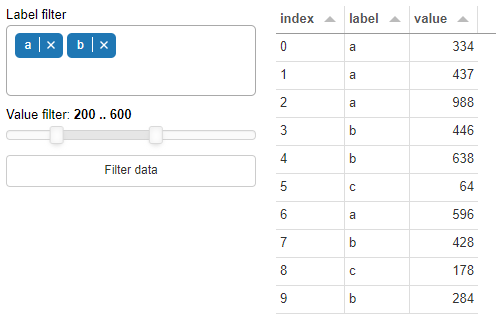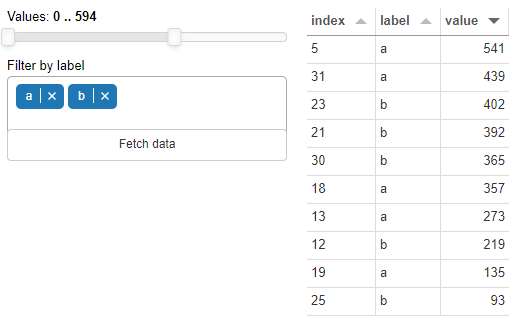# How to get a decent understanding of param.Parameterized classes?

Hello All!

I am trying to make a simple dashboard, where I have some filters and a button, which when pressed should show the DataFrame with the filtered data.

Something in line with this, for instance:I feel that such a dashboard is a good use case for param.Parameterized classes, where `@param.depends('button')` would trigger the Data to update and therefore I wished to get a decent understanding of how it all works.

However, I feel somehow stuck in getting started. Probably because I am not sure what would be the best practices to use pn.widgets.MultiChoice within param.Parameterized.

The following code seems to work, although I am not fully sure why.
I am still skeptical that I am doing it correctly and I will not run into issues later on.

``````import panel as pn
import param
import pandas as pd
import random

df = pd.DataFrame(
data={
'label': [random.choice(list('abc')) for _ in range(100)],
'value': random.sample(range(1, 1000), 100)
}
)

LABELS = list(df['label'].unique())

class FilterData(param.Parameterized):
max_value = df['value'].max()
values = param.Range(default=(0, max_value), bounds=(0, max_value), step=max_value // 10)
labels = pn.widgets.MultiChoice(value=LABELS, name="Filter by label", options=LABELS)
button = param.Action(lambda x: x.param.trigger('button'), label='Fetch data')

@param.depends('button')
def output_panel(self):
df_panel = pn.widgets.Tabulator(df[(df['value'] >=  self.values) & (df['value'] <=  self.values) & (df['label'].isin(self.labels.value))].head(10))
return df_panel

def dashboard(self):
dashboard = pn.Row(
pn.Column(
self.param['values'],
self.labels,
self.param['button'],
width=300
),
self.output_panel
)
return dashboard

filter = FilterData()
filter.dashboard().show()
``````Also, I am not sure why the MultiChoice component goes into the button…
I don’t think I can mark my own answer as the solution.

You are almost there, though some small tips:

• You should almost never use a `pn.widgets` in the class declaration, as all objects will share it.
• Instead, you should use `param.Parameter` and `pn.Param` either inside the class or outside on the object. I have chosen the first in my example below.
• Try to look at `pn.viewable.Viewer`, which is almost the same as a `param.Parameterized` class except it has a `__panel__` method which removes the need for calling your `dashboard` method. (I haven’t done that in my example below).
``````class FilterData(param.Parameterized):
max_value = df['value'].max()
values = param.Range(default=(0, max_value), bounds=(0, max_value), step=max_value // 10)
labels = param.ListSelector(default=LABELS, objects=LABELS)
button = param.Action(lambda x: x.param.trigger('button'), label='Fetch data')

@param.depends('button')
def output_panel(self):
df_panel = pn.widgets.Tabulator(df[(df['value'] >=  self.values) & (df['value'] <=  self.values) & (df['label'].isin(self.labels))].head(10))
return df_panel

def dashboard(self):
sidebar = pn.Param(self.param, widgets={"labels": pn.widgets.MultiChoice}, width=300)

dashboard = pn.Row(
sidebar,
self.output_panel
)
return dashboard
``````
2 Likes

Thank you for the tips, @Hoxbro
For some reason I can’t mark your answer as solution - I don’t even see the solution checkbox.

2 Likes

I believe that marking a post as a solution has not been enabled for the param category? I am not an discourse admin and cannot do it.

Can you do it @droumis? See GitHub - discourse/discourse-solved: Allow accepted answers on topics. If you can do it please enable it across all categories. Thanks.

1 Like

done and marked

2 Likes